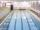# Railway

Railway line had on 5.8 km segment climb 9 permille. How many meters track ascent?

Result

x =  52.198 m

#### Solution:Leave us a comment of example and its solution (i.e. if it is still somewhat unclear...):

Showing 0 comments:Be the first to comment!#### To solve this example are needed these knowledge from mathematics:

Our permille calculator will help you quickly calculate various typical tasks with permilles. See also our right triangle calculator. See also our trigonometric triangle calculator.

## Next similar examples:

1. LiftThe largest angle at which the lift rises is 16°31'. Give climb angle in permille.
2. ClimbFor horizontal distance 4.9 km road rise by 6.1 m. Calculate the road pitch in ‰ (permille, parts per thousand).
3. RoadAverage climb of the road is given by ratio 1:15. By what angle road average climb?
4. High wallI have a wall 2m high. I need a 15 degree angle (upward) to second wall 4 meters away. How high must the second wall?
5. TreeHow tall is the tree that observed in the visual angle of 52°? If I stand 5 m from the tree and eyes are two meters above the ground.
6. Cable car 2Cable car rises at an angle 41° and connects the upper and lower station with an altitude difference of 1175 m. How long is the track of cable car?
7. 30-60-90The longer leg of a 30°-60°-90° triangle measures 5. What is the length of the shorter leg?
8. Center traverseIt is true that the middle traverse bisects the triangle?
9. Reference angleFind the reference angle of each angle:
10. Height differenceWhat height difference overcome if we pass road 1 km long with a pitch21 per mille?
11. Height 2Calculate the height of the equilateral triangle with side 38.
12. MapleMaple peak is visible from a distance 3 m from the trunk from a height of 1.8 m at angle 62°. Determine the height of the maple.
13. SinesIn ▵ ABC, if sin(α)=0.5 and sin(β)=0.6 calculate sin(γ)
14. TrigonometryIs true equality? ?
15. ReflectorCircular reflector throws light cone with a vertex angle 49° and is on 33 m height tower. The axis of the light beam has with the axis of the tower angle 30°. What is the maximum length of the illuminated horizontal plane?
16. Theorem proveWe want to prove the sentense: If the natural number n is divisible by six, then n is divisible by three. From what assumption we started?
17. Slope of the poolCalculate slope (rise:run) of the bottom of swimming pool long 10 m. Water depth at beginning of pool is 1.16 m (for children) and depth at end is 1.89 m (for swimmers). Slope express as percentage and as angle in degrees.